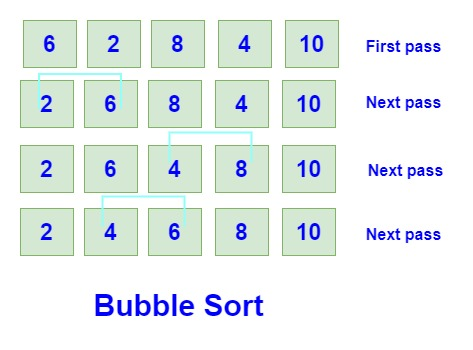# Bubble sort algorithm in java

## What is bubble sort?Bubble Sort is one of the most common sorting algorithms used to sort small datasets.  It is a straightforward algorithm. It does the internal sorting means it does not require auxiliary memory like merge sort to do the sorting operation.

What bubble sort do is compare the adjacent element and if the adjacent element (on the right side) is smaller then it performs the swapping operation.

## Implementation of the bubble sort algorithm

Create a method with the name bSort (you can take any name) which takes integer array  a1[]  as input.

Then we calculate the length of a given array and stored it in a variable n.

Now we will create a nested for loop to iterate over the array and perform the sorting operation. Outer for loop will iterate over all elements in the array which we will call pass.

Then inner for loop or nested for loop will start from the first element of the array a1[i] till the last element n-i-1.

Inside the nested loop, there will be the condition to check for the adjacent smaller number  a1[j] > a1[j+1]

If the adjacent number on the right side is smaller than the current number then it will perform the swapping operation to shift the smaller number to the right side.

In this way, we will get the largest element in the array at the position of n-1 and the smallest number of the array at the beginning.

## Bubble sort in java

``````public class BubbleSort {

static void bSort(int a1[]){

int n = a1.length;

for(int i=0; i<n-1; i++){

for(int j=0; j<n-i-1; j++){

if(a1[j] > a1[j+1]){ /// < for descending

int temp = a1[j];
a1[j] = a1[j+1];
a1[j+1] = temp;
}
}
}
}

static void display(int a1[]){

for(int x : a1){

System.out.print(x+" ");
}
}

public static void main(String args[]){

int a1[] = {6, 2, 8, 4, 10};
bSort(a1);
display(a1);
}
}
``````

## Descending order / reverse sort using bubble sort

In case you asked to sort the elements in descending order, then you just have to change the simple condition.

To sort in descending order using bubble sort => a1[j] < a1[j+1]

That’s it! straight forward!

## Time complexity of bubble sort

Though bubble sort is easy to apply it does not give us the best results in terms of time complexity. It is one of the worst algorithms in terms of time complexity. As it is using nested for loop and it iterates over each and every element though they are fully or partially sorted.

Best Time Complexity: Ω(n)

Average Time Complexity:  θ(n^2)

Worst Time Complexity: O(n^2)

## The space complexity of bubble sort

In space complexity, we always consider the worst complexity. So in the case of bubble sort, it will be O(1).